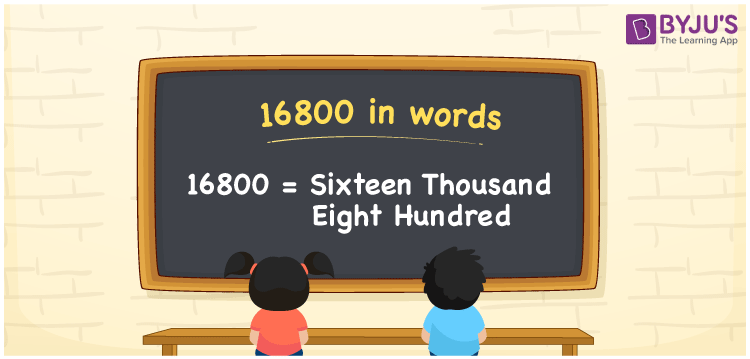# 16800 in Words

16800 in words can be written as Sixteen thousand eight hundred . This article will provide a strong knowledge of the concept of counting. For instance, if you buy a TV for Rs. 16800 at the store, then you can say that “I bought a TV for Sixteen thousand eight hundred  Rupees at the store”. Students will also learn how to write numbers in words using the English alphabet. Hence, the number 16800 in words can be read as “Sixteen thousand eight hundred ”.

 16800 in words Sixteen thousand eight hundred Sixteen thousand eight hundred  in Numbers 16800

## 16800 in English Words## How to Write 16800 in Words?

Below you will learn about the place value chart for 16800 in words with the help of the table. 16800 has five digits and the place value for each digit can be understood easily by referring to the table here.

 Ten Thousands Thousands Hundreds Tens Ones 1 6 8 0 0

16800 in expanded form is:

1 x Ten Thousand + 6 x Thousand + 8 × Hundred + 0 × Ten + 0 × One

= 1 x 10000 + 6 x 1000 + 8 × 100 + 0 × 10 + 0 × 1

= 10000 + 6000 + 800

= 16800

= Sixteen thousand eight hundred

Therefore, 16800 in words is written as Sixteen thousand eight hundred .

16800 is a natural number that precedes 16801 and succeeds 16799.

16800 in words – Sixteen thousand eight hundred

Is 16800 an odd number? – No

Is 16800 an even number? – Yes

Is 16800 a perfect square number? – No

Is 16800 a perfect cube number? – No

Is 16800 a prime number? – No

Is 16800 a composite number? – Yes

## Frequently Asked Questions on 16800 in Words

### How do you write 16800 in words?

16800 can be written as “Sixteen thousand eight hundred ” in words.

### Determine the value of 17000 minus 200.

The value of 17000 minus 200 is 16800. Therefore, 16800 in words is Sixteen thousand eight hundred.

### Is 16800 an odd or even number?

16800 is an odd number as it is completely divisible by 2.
16800/2 = 8400